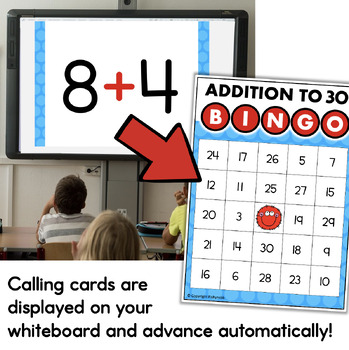DID YOU KNOW:
Seamlessly assign resources as digital activities

Learn how in 5 minutes with a tutorial resource. Try it Now

Learn More# 1st Grade Math Bingo Games for Powerpoint BUNDLE1st - 2nd
Subjects
Standards
Resource Type
Formats Included
• Zip
Pages
180 pages
\$9.75
Bundle
List Price:
\$13.00
You Save:
\$3.25
\$9.75
Bundle
List Price:
\$13.00
You Save:
\$3.25

### Description

This is a bundle of first grade math games for Powerpoint. They run automatically, so it's very little work for the teacher!

INCLUDES

• 10 More 10 Less Bingo Game
• Addition Within 30 Bingo Game
• Place Value to 50 Bingo Game
• Telling Time to the Half Hour Bingo Game

Each game includes 22-24 Bingo cards plus a Powerpoint presentation for playing the game. The Powerpoint is designed to run itself. Just click the LET'S PLAY button and the slides will advance automatically. As the game runs, students solve the problems and mark the matching answers on their Bingo cards.

These games are a fun way to build number sense and math fluency! They are very easy to use and allow the teacher to work on other things or help students while they play. Great for sub days too!

FIND MORE GAMES HERE:

BINGO Games for Powerpoint

Total Pages
180 pages
N/A
Teaching Duration
N/A
Report this Resource to TpT
Reported resources will be reviewed by our team. Report this resource to let us know if this resource violates TpT’s content guidelines.

### Standards

to see state-specific standards (only available in the US).
Fluently add and subtract within 20 using mental strategies. By end of Grade 2, know from memory all sums of two one-digit numbers.
Add and subtract within 20, demonstrating fluency for addition and subtraction within 10. Use strategies such as counting on; making ten (e.g., 8 + 6 = 8 + 2 + 4 = 10 + 4 = 14); decomposing a number leading to a ten (e.g., 13 - 4 = 13 - 3 - 1 = 10 - 1 = 9); using the relationship between addition and subtraction (e.g., knowing that 8 + 4 = 12, one knows 12 - 8 = 4); and creating equivalent but easier or known sums (e.g., adding 6 + 7 by creating the known equivalent 6 + 6 + 1 = 12 + 1 = 13).
Fluently add and subtract within 100 using strategies based on place value, properties of operations, and/or the relationship between addition and subtraction.
Given a two-digit number, mentally find 10 more or 10 less than the number, without having to count; explain the reasoning used.
Add within 100, including adding a two-digit number and a one-digit number, and adding a two-digit number and a multiple of 10, using concrete models or drawings and strategies based on place value, properties of operations, and/or the relationship between addition and subtraction; relate the strategy to a written method and explain the reasoning used. Understand that in adding two-digit numbers, one adds tens and tens, ones and ones; and sometimes it is necessary to compose a ten.